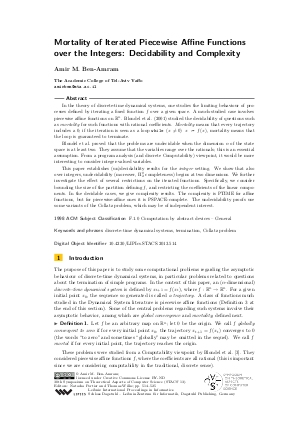Document# Mortality of Iterated Piecewise Affine Functions over the Integers: Decidability and Complexity (Extended Abstract)

### Author Amir M. Ben-Amram## File

LIPIcs.STACS.2013.514.pdf
• Filesize: 0.57 MB
• 12 pages

## Cite As

Amir M. Ben-Amram. Mortality of Iterated Piecewise Affine Functions over the Integers: Decidability and Complexity (Extended Abstract). In 30th International Symposium on Theoretical Aspects of Computer Science (STACS 2013). Leibniz International Proceedings in Informatics (LIPIcs), Volume 20, pp. 514-525, Schloss Dagstuhl - Leibniz-Zentrum für Informatik (2013)
https://doi.org/10.4230/LIPIcs.STACS.2013.514

## Abstract

In the theory of discrete-time dynamical systems, one studies the limiting behaviour of processes defined by iterating a fixed function f over a given space. A much-studied case involves piecewise affine functions on R^n. Blondel et al. (2001) studied the decidability of questions such as mortality for such functions with rational coefficients. Mortality means that every trajectory includes a 0; if the iteration is seen as a loop while (x \ne 0) x := f(x), mortality means that the loop is guaranteed to terminate. Blondel et al. proved that the problems are undecidable when the dimension n of the state space is at least two. They assume that the variables range over the rationals; this is an essential assumption. From a program analysis (and discrete Computability) viewpoint, it would be more interesting to consider integer-valued variables. This paper establishes (un)decidability results for the integer setting. We show that also over integers, undecidability (moreover, Pi^0_2 completeness) begins at two dimensions. We further investigate the effect of several restrictions on the iterated functions. Specifically, we consider bounding the size of the partition defining f, and restricting the coefficients of the linear components. In the decidable cases, we give complexity results. The complexity is PTIME for affine functions, but for piecewise-affine ones it is PSPACE-complete. The undecidability proofs use some variants of the Collatz problem, which may be of independent interest.
##### Keywords
• discrete-time dynamical systems
• termination
• Collatz problem

## Metrics

• Access Statistics
• Total Accesses (updated on a weekly basis)
0